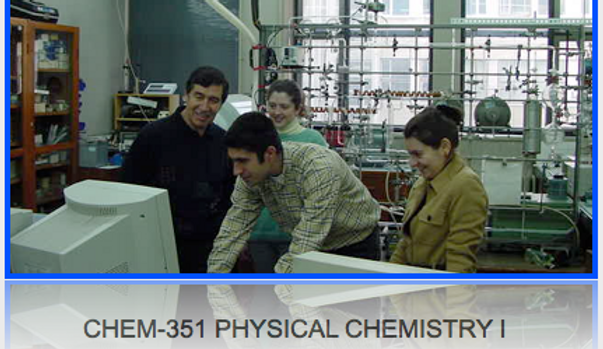top of page

Physical chemistry (Chem-351) is a required course in undergraduate curricula of the Chemistry Department. Physical chemistry is fundamental to chemistry. It is therefore can be defined as "that part of chemistry which is fundamental, molecular and interesting". The first course in physical chemistry should not include a little bit of everything in the field of physical chemistry. Instead, to my opinion, it should concentrate on fundamentals and their applications in depth. The foundation of physical chemistry is quantum mechanics then follows statistical mechanics, thermodynamics and kinetics.

1.Quantum Mechanics: The mechanics of atoms and the mechanics of their combinations in molecules.

2.Statistical Mechanics: The framework by which molecular properties can be related to the macroscopic behaviour of chemical substances.

3.Thermodynamics: The study of energy and entropy, and their connections with chemical changes and chemical equilibrium.

4.Kinetics: The study of rates of chemical reactions and the study of molecular processes by which reactions occurs.

Mathematics is the biggest difficulty for students beginning their study of physical chemistry. It is therefore math background is very important. Algebra, analytical geometry, and calculus are unavoidable in this respect. Before beginning this course fresh your mathematical background in basic calculus including power series, curve fitting, and multivariable partial differentiation. You should be able to differentiate simple functions easily and should form differentials. This should provide a first insight of the mathematics needed with the material in this course. Read chapter material as presented in syllabus part before coming the lecture in order to get the direction of the presentation and a general feel for the subtopics. Another difficulty in the path of learning physical chemistry is to memorize equations. Memory should be reserved for the fundamentals and important definitions. Equations are meant to be understood, not to be memorized. A physical chemical equation is not a jumbled mass of symbol, but is a statement of a relation between physical quantities. After each lecture presentation on some topic or subtopic read corresponding text sections from your textbook consulting lecture handouts and your lecture notes. Understand all mathematical steps and go over the examples given in lectures. One of the most important learning process in physical chemistry is working exercises. Working on exercises is a must for understanding physical chemistry. Exercises will help your understanding of newly introduced quantities and functions. Get the habits working with other students. Especially try to devise your own exercises with your own solutions, and then try to eplain to them to other students.bottom of page